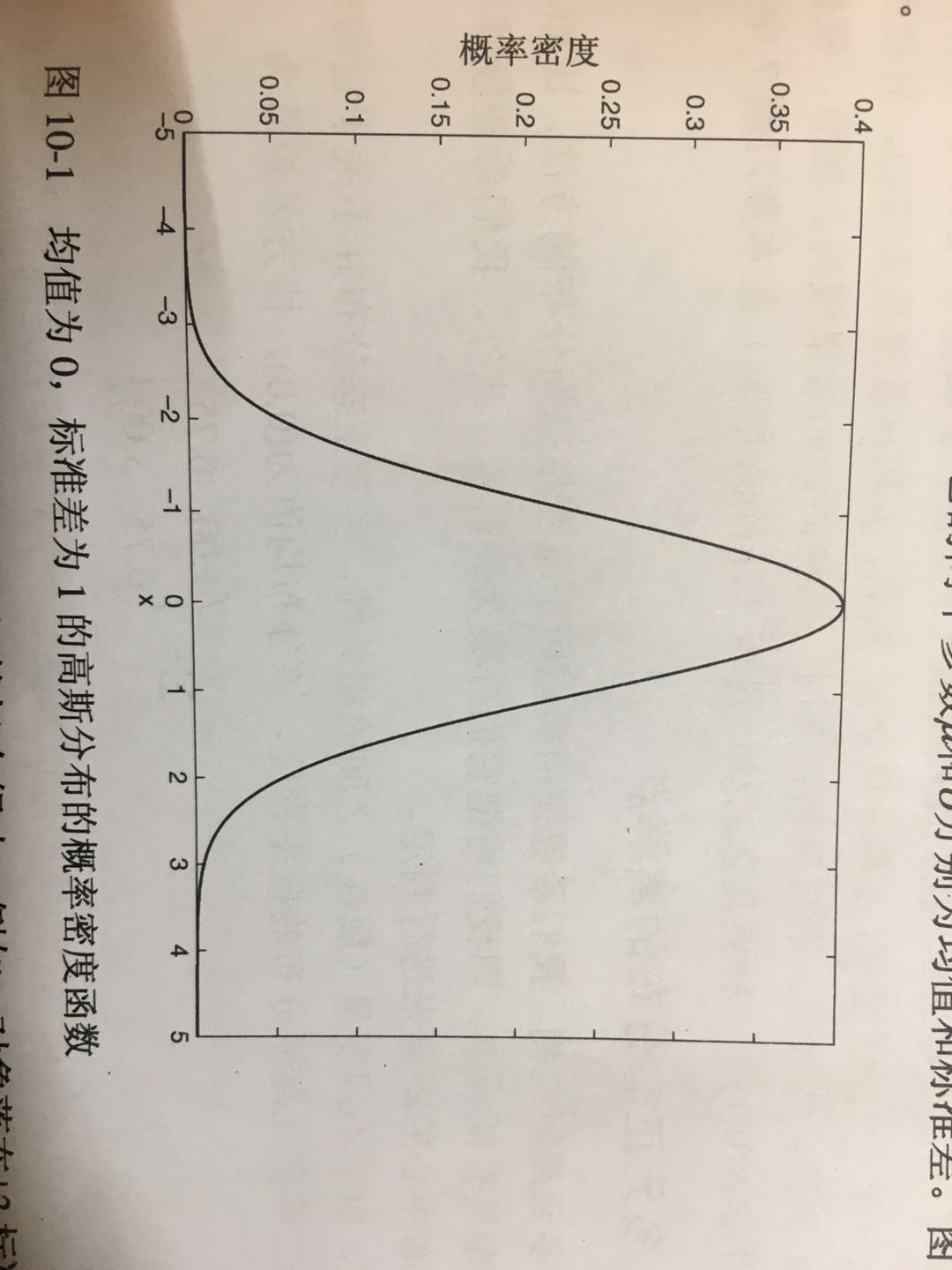• 参数估计就是根据样本统计量的数值对总体参数进行估计的过程。根据参数估计的性质不同，可以分成两种类型：点估计和区间估计。 点估计 点估计就是用样本统计量的某一具体...对总体参数进行点估计常用方法有两种...
参数估计就是根据样本统计量的数值对总体参数进行估计的过程。根据参数估计的性质不同，可以分成两种类型：点估计和区间估计。
点估计
点估计就是用样本统计量的某一具体数值直接推断未知的总体参数。例如，在进行有关小学生身高的研究中，随机抽取1000名小学生并计算出他们的平均身高为1.46米。如果直接用这个1.46米代表所有小学生的平均身高，那么这种估计方法就是点估计。
对总体参数进行点估计常用的方法有两种：矩估计与最大似然估计，其中最大似然估计就是我们实际中使用非常广泛的一种方法。 按这两种方法对总体参数进行点估计，能够得到相对准确的结果。如用样本均值X估计总体均值，或者用样本标准差S估计总体标准差σ。
点估计有一个不足之处，即这种估计方法不能提供估计参数的估计误差大小。对于一个总体来说，它的总体参数是一个常数值，而它的样本统计量却是随机变量。当用随机变量去估计常数值时，误差是不可避免的，只用一个样本数值去估计总体参数是要冒很大风险的。因为这种误差风险的存在，并且风险的大小还未知，所以，点估计主要为许多定性研究提供一定的参考数据，或在对总体参数要求不精确时使用，而在需要用精确总体参数的数据进行决策时则很少使用。
区间估计
区间估计就是在推断总体参数时，还要根据统计量的抽样分布特征，估计出总体参数的一个区间，而不是一个数值，并同时给出总体参数落在这一区间的可能性大小，概率的保证。还是举小学生身高的例子，如果用区间估计的方法推断小学生身高，则会给出以下的表达：根据样本数据，估计小学生的平均身高在1.4~1.5米之间，置信程度为95%，这种估计就属于区间估计。


展开全文统计学
• 离群检测常用方法 一、基于统计方法 统计学方法是基于模型方法，其首先给待检测数据集预设一个模型，然后将数据集中对象与预设模型拟合程度来检测数据集中离群。基于统计学离群检测方法大多都基于...
离群点检测常用方法

一、基于统计的方法

统计学方法是基于模型的方法，其首先给待检测数据集预设一个模型，然后将数据集中对象与预设模型的拟合程度来检测数据集中的离群点。基于统计学的离群点检测方法大多都基于构建一个概率分布模型，并考虑数据集中对象与该概率分布模型相符合的程度。

定义1.基于统计学方法的离群点定义

离群点是一个对象，关于数据的概率分布模型，它具有低概率。

概率分布模型通过估计用户指定的分布参数，由数据创建。例如如果某数据分布满足高斯分布或者泊松分布，则其基本分布的均值和标准差均可以通过计算数据的均值和标准差来估计。然后可以估计每个对象在该分布下的概率。在统计学术语中，离群点又被称为“不和谐的观测值（discordant observation）”

基于统计学的离群点检测方法的主要问题在于：虽然许多类型的数据都可以用诸如高斯分布、泊松分布或二项式分布等描述，但是不满足常见分布的数据集也很多。如果针对待检测数据集建立了错误的预分布模型，则很可能将数据集中的正常对象误判为离群点。例如数据有可能来自于满足高斯分布的模型，但却错误的将其建模为另一种分布，使得其相比于高斯分布更远的偏离与均值。这类行为的统计分布在实践中常被称为重尾分布（heavy-tailed distribution）。

大部分基于统计学的离群点检测算法都使用单个属性，但是目前已经有了混合分布的检测方法。基于混合分布的检测方法对数据建模，虽然可能功能更加强大，但这种混合分布的模型更加复杂，较难理解和使用。

1.1  一元正态分布中的离群点检测

高斯分布或者称正态分布是统计学中最为常见的一种分布，用N（，）表示高斯分布，其中表示均值，表示方差。如图所示为均值为0，标准差为1的概率密度函数。如果数据集满足该模型分布，那么该数据集中的绝大多数数据点将聚集在（-3,3）之间，而落在这区间之外的数据对象个数很少，概率仅有0.0027。用形式化语言描述如下：

如果c是常数，x表示数据集中的属性值，则||x||>=c的概率随着c增大而迅速减小。设=probability(|x|>=c)，

展开全文数据挖掘
• 文章目录1、前言2、最大似然估计法 MLE3、最大后验估计 MAP4、贝叶斯估计5、其他的参数估计方法 1、前言 我们讨论的是有参的情况，在这种情况中，...而 ML 中主要是构造点估计的方法常用的有：①最大似然估计法，...
文章目录1、前言2、最大似然估计法 MLE3、最大后验估计 MAP4、贝叶斯估计5、其他的参数估计方法
1、前言
我们讨论的是有参的情况，在这种情况中，我们的目标是估计参数值（假设有可能确定真是参数），而不是函数值。在概率论中，参数估计有点估计(point estimation)和区间估计(interval estimation)两种。而 ML 中主要是构造点估计的方法常用的有：①最大似然估计法，用来求一个样本集的相关概率密度函数的参数；②最小二乘法，主要用于线性统计模型中的参数估计问题；③贝叶斯估计法；等等。
2、最大似然估计法 MLE
MLE（maximum likelihood estimation）通常是机器学习中首选的估计方法，因为它具有一致性（当训练样本数目趋向无穷大时，参数的最大似然估计就会收敛到参数的真实值） 和 统计效率（输入与输出存在不平衡的关系）。
最大似然参数求解的核心思想就是构造当前样本出现的联合概率函数，对其求偏导，让当前样本的概率最大的就是模型参数。
考虑一组含有 m 个样本的数据集X=$x_1,x_2,...,x_m\brace$ ，独立地由未知的真实数据生成分别$p_{data} (x)$生成。令 $p_{model} (x;\theta)$是一族由$\theta$确定的相同空间上的概率分布。对$\theta$的MLE被定义为：
$\theta_{ML} = \arg\max_{\theta}p_{model} (X;\theta) =\arg\max_{\theta}\prod_{i}^{m} p(x_i;\theta) \tag{1}$求解过程：通常将乘积转成求和（乘积会导致在计算中出现数值下溢），也就是取对数，然后再进行求偏导等操作。
$\theta_{ML} = \arg\max_{\theta} \sum_{i}^{m} \log p(x_i;\theta) \tag{2}$
和MLE类似的参数估计方法还有：

条件对数似然：估计条件概率$p(y|x;\theta)$，从给定x预测y:$\theta_{ML} = \arg\max_{\theta} \sum_{i}^{m} \log p(y_i|x_i;\theta) \tag{3}$
KL散度

3、最大后验估计 MAP
MAP 估计选择后验概率最大的点（或在$\theta$是连续纸的更常见的情况下，概率密度最大的点）为最终的模型参数。$\theta_{MAP} = \arg\max_{\theta}p(\theta|x)= \arg\max_{\theta}\log p(x|\theta) +\log p(\theta) \tag{4}$可以看出右项中$\log p(x|\theta)$对应这标准的对数似然，$\log p(\theta)$对应着先验分布。

MAP增加了先验信息，有助于减少最大后验估计的方差，但是也增加了偏差；
具有高斯先验权重的MAP贝叶斯推断对应着权重衰减，此时先验项$\log p(\theta)$正比于权重衰减惩罚$\lambda w^Tw$ (MAP提供了一个直观的方法来设计复杂但可解释的正则化项)；权重衰减正则化的最大似然学习，就可以解释为贝叶斯推断的MAP近似。
MLE和MAP都是估计单一值$\theta$的方法，但是MLE是频率派统计方法，而MAP是贝叶斯派方法。

4、贝叶斯估计
贝叶斯估计是在MAP上做进一步拓展，此时不直接估计参数的值，而是允许参数服从一定概率分布（MLE和MAP都是估计单一值$\theta$的方法）。
贝叶斯估计通过贝叶斯规则结合数据似然和先验，可以恢复数据对我们关于$\theta$的信念的影响：$p(\theta|x_1,x_2,...,x_m)=\frac{p(x_1,x_2,...,x_m|\theta)p(\theta)}{p(x_1,x_2,...,x_m)}$
预测：$p(x_{m+1}|x_1,x_2,...,x_m)=\int{p(x_{m+1}|\theta)p(\theta|x_1,x_2,...,x_m)}d_{\theta}$

预测时，贝叶斯估计使用的$\theta$的全分布
当训练数据有限时，贝叶斯方法通常泛化得更好，当数据量很大时，通常会有很大的计算代价。

5、其他的参数估计方法

求解算法的模型参数的常用方法
适用范围
过程
优点
缺点
其他

OLS
用来做函数拟合或者求函数极值的方法（在机器学习，尤其是回归模型中，常用）
1、 线性：$\red{\theta=(X^TX)^{-1}X^TY}$2、 非线性：迭代法
适用简洁高，  不需要选择步长， 也不用迭代求解，  最小二乘法是计算解析解。
$1. \red{X^TX 要可逆}$，            2. 如果样本量很大，用最小二乘法由于需要求一个超级大的逆矩阵，这时就很难或者很慢才能求解解析解了，使用迭代的梯度下降法比较有优势。                                     3. 拟合函数需要是线性的

带权的最小二乘（WLS，IRLS）
用于线性回归、逻辑回归、AFT生存回归和多层感知器的求解
1、线性化目前的解决方案的目标，并更新相应的权重。2、通过Weighted Least Squares解决加权最小二乘（WLS）问题。3、重复上述步骤直到收敛。
加入了权重矩阵，进一步提高状态估计精度
为了使正则方程逼近是有效的，加权最小二乘要求特征的数量不超过4096个。  对于规模更大的问题，是有L-BFGS。

Gradient Descent  （BGD，SGD，MBGD
在最小化损失函数时，可以通过梯度下降法来一步步的迭代求解，得到最小化的损失函数，和模型参数值。
1、根据miniBatch Fraction参数进行样本抽样，获得一个小样本集;    2、调用Gradient计算在小样本集上的梯度值；  3、调用Updater,根据regParam、stepSize、numIterations等参数值更新模型参数；  4、判断终止条件(精度收敛或者迭代次数达到上限)，否则继续上面步骤。
迭代求解，速度较快
需选择步长和初值；  局部最优解（梯度下降可以逼近解析解，要保证你的训练数据是凸）
梯度下降法和最小二乘法相比：  梯度下降法需要选择步长，而最小二乘法不需要。  梯度下降法是迭代求解，最小二乘法是计算解析解。  如果样本量不算很大，且存在解析解，最小二乘法比起梯度下降法要有优势，计算速度很快。  但是如果样本量很大，用最小二乘法由于需要求一个超级大的逆矩阵，这时就很难或者很慢才能求解解析解了，使用迭代的梯度下降法比较有优势。

牛顿法
非线性逼近
解方程组→得到搜索方向→移动点→检验收敛条件
收敛快，二阶收敛，因此它比最速下降法要快
需要计算嗨森矩阵，对一般问题不是整体收敛的，只有当初始点充分接近极小点时，才有很好的收敛性，  牛顿法的鲁棒性较差（H要正定）
梯度下降法和牛顿法/拟牛顿法相比:  两者都是迭代求解，不过梯度下降法是梯度求解，而牛顿法/拟牛顿法是用二阶的海森矩阵的逆矩阵或伪逆矩阵求解。  相对而言，使用牛顿法/拟牛顿法收敛更快。但是每次迭代的时间比梯度下降法长。

拟牛顿法（lbfgs
非线性逼近
算法只保存并利用最近m次迭代的曲率信息来构造海森矩阵的近似矩阵。
收敛速度介于梯度下降法和牛顿法之间，是超线性的。  L-BFGS方法以二次方程来逼近目标函数来构造黑塞矩阵，不考虑目标函数的二阶偏导数。  L-BFGS通常比其他一阶优化方法能更快收敛。每次迭代的开销非常小，每一步迭代都能保证近似矩阵的正定，因此算法的鲁棒性还是很强的。


展开全文机器学习
• 本文选择几种常用的点估计方法作一些讨论。 用于估计未知参数的统计量称为点估计（量）。参数 θθ\theta 估计量常用 θ^=θ^(x1,x2,…,xn)θ^=θ^(x1,x2,…,xn)\hat{\theta} = \hat{\theta}(x_{1},x_{2}, \dots,...
参数估计有两种形式：点估计与区间估计。本文选择几种常用的点估计方法作一些讨论。
用于估计未知参数的统计量称为点估计（量）。参数 $\theta$ 的估计量常用 $\hat{\theta} = \hat{\theta}(x_{1},x_{2}, \dots, x_{n})$ 表示，参数 $\theta$ 的可能取值范围称为参数空间，记为 $\Theta = \{\theta\}$。
文章目录最大似然估计贝叶斯估计
最大似然估计
最大似然估计，即对似然函数最大化，其关键是从样本 $x$ 和含有位置参数 $\theta$ 的分布 $p(x,\theta)$ 获得似然函数。设 $x=(x_{1},x_{2},\dots,x_{n})$ 是来自含有未知参数的某分布 $p(x,\theta)$ 的一个样本，那么其联合分布为：
$p(x,\theta) = \prod_{i=1}^{n}p(x_{i},\theta)$ 其中 $p(x_{i},\theta)$ 在连续场合是指密度函数在 $x_{i}$ 处的值，在离散场合为分布列中的一个概率 $P_{\theta}(X=x_{i})$ 。对样本分布 $p(x,\theta)$ 我们知道：

样本如何产生？先有 $\theta$ 后有 $x$，即先有一个给定的 $\theta$ 的值 $\theta_{0}$，然后由分布 $p(x,\theta_{0})$ 经过随机抽样产生样本观察值 $x$。
如今我们有了 $x$ 如何追溯参数 $\theta_{0}$ 呢？当给定样本观察值 $x$ 时样本分布 $p(x,\theta)$ 仅是 $\theta$ 的函数，可记为 $L(\theta,x)$ 或 $L(\theta)$，并称其为似然函数。对于不同的 $\theta_{1},\theta_{2}\in\Theta$，可使得样本观察值 $x$ 出现的机会不同。若 $L(\theta_{1}) > L(\theta_{2})$，表明 $\theta_{1}$ 会使 $x$ 出现的机会比 $\theta_{2}$ 更大些，即 $\theta_{1}$ 比 $\theta_{2}$ 更像真值 $\theta_{0}$。也就是说 $L(\theta)$ 成为了度量 $\theta$ 更像真值的程度，其值越大越像。按此思路，在参数空间 $\Theta$ 中使 $L(\theta)$ 最大的 $\hat{\theta}$ 就是最像 $\theta_{0}$ 的真值，这个 $\hat{\theta}$ 就是 $\theta$ 的最大似然估计。

这里给出两个实例。
1.伯努利分布实例
假设 $P(X=1)=p,P(X=0)=1-p$ 综合起来就有
$P(X)=p^{X}(1-p)^{1-X}$
此时如果有一组数据 $D$ 是从这个随机变量中采样得到的，那么就有
\begin{aligned} \ max_{p}\log P(D)&= \max_{p}\log\prod_{i}^{N}P(D_{i}) \\ &=\max_{p}\sum_{i}^{N}\log P(D_{i}) \\ &=\max_{p}\sum_{i}^{N}[D_{i}\log p+(1-D_{i})\log(1-p)] \end{aligned}
对上式求导，则有
$\nabla_{p}\max_{p}\log P(D)=\sum_{i}^{N}[D_{i}\frac{1}{p}+(1-D_{i})\frac{1}{p-1}]$
求极值，令导数为 $0$，就有
\begin{aligned} & \sum_{i}^{N}[D_{i}\frac{1}{p}+(1-D_{i})\frac{1}{p-1}]=0 \\ & \sum_{i}^{N}[D_{i}(p-1)+(1-D_{i})p]=0 \\ & \sum_{i}^{N}(p-D_{i})=0 \\ & p=\frac{1}{N}\sum_{i}^{N}D_{i} \end{aligned}
即全部采样的平均值。
2.高斯分布实例
令 $p(x)=\frac{1}{\sqrt{2\pi\sigma^{2}}}e^{-\frac{(x-\mu)^{2}}{2\sigma^{2}}}$，采用同样的方法有
\begin{aligned} \max_{p}\log P(D) &= \max_{p}\log\prod_{i}^{N}P(D_{i}) \\ &= \max_{p}\sum_{i}^{N}\log P(D_{i}) \\ &= \max_{p}\sum_{i}^{N}[-\frac{1}{2}\log(2\pi\sigma^{2})-\frac{(D_{i}-\mu)^{2}}{2\sigma^{2}}] \\ &= \max[-\frac{N}{2}\log(2\pi\sigma^{2})-\frac{1}{2\sigma^{2}}\sum_{i}^{N}(D_{i}-\mu)^{2}] \end{aligned}
此处包含两个参数，分别估计。
首先对 $\mu$ 求导，有
$\frac{\partial\max_{\mu}\log P(D)}{\partial \mu} = -\frac{1}{\sigma^{2}}\sum_{i}^{N}(\mu-D_{i})$
令导数为 $0$，有
$-\frac{1}{\sigma^{2}}\sum_{i}^{N}(\mu-D_{i})=0,\quad \mu=\frac{1}{N}\sum_{i}^{N}D_{i}$
注意很容易看出这个结果与最小二乘计算完全相同，实质上最小二乘法可以与极大似然法中假定误差遵循正态分布的特殊情况相对应。
其次对 $\sigma^{2}$ 求导，有
$\frac{\partial\max_{\sigma^{2}}\log P(D)}{\partial\sigma^{2}} = -\frac{N}{2\sigma^{2}}+\frac{1}{2\sigma^{4}}\sum_{i}^{N}(D_{i}-\mu)^{2}$
令导数为 $0$，有
$-\frac{N}{2\sigma^{2}}+\frac{1}{4\sigma^{4}}\sum_{i}^{N}(D_{i}-\mu)^{2}=0$
$\sigma^{2} = \frac{1}{N}\sum_{i}^{N}(D_{i}-\mu)^{2}$
可见最终计算结果与期望方差计算方式完全一致。注意最大似然估计并不一定具有无偏性。
对似然函数添加或剔除一个与参数 $\theta$ 无关的量 $c(x)>0$，不影响寻求最大似然估计的最终结果，故 $c(x)L(\theta)$ 仍然是 $\theta$ 的似然函数。例如，对于正态分布而言：
$L(\mu,\sigma^{2}) = \prod_{i=1}^{n}\frac{1}{\sqrt{2\pi}\sigma^{2}}e^{-\frac{(x_{i}-\mu)^{2}}{2\sigma^{2}}} \propto (\sigma^{2})^{-\frac{n}{2}}\exp\left\{-\frac{1}{2\sigma^{2}}\sum_{i=1}^{n}(x_{i}-\mu)^{2}\right\}$
不变原理： 设 $X\sim p(x,\theta), \theta\in\Theta$，若 $\theta$ 的最大似然估计为 $\hat{\theta}$ ，则对任意函数 $\gamma=g(\theta)$，$\gamma$ 的最大似然估计为 $\hat{\gamma}=g(\hat{\theta})$。
贝叶斯估计
统计学中有两个主要学派：频率学派（又称经典学派）和贝叶斯学派。前述最大似然估计属于经典统计学范畴。频率学派利用总体信息和样本信息进行统计推断，贝叶斯学派与之的区别在于还用到了先验信息。
贝叶斯学派最基本的观点是：任一未知量 $\theta$ 都可以看做随机变量，可用一个概率分布区描述，这个分布称为先验分布 （记为 $\pi(\theta)$）。因为任一未知量都有不确定性，而在表述不确定性地程度时，概率与概率分布是最好的语言。依赖于参数 $\theta$ 的密度函数在经典统计学中记为 $p(x,\theta)$，它表示参数空间 $\Theta$ 中不同的 $\theta$ 对应不同的分布。在贝叶斯统计中应记为 $p(x|\theta)$ ，表示随机变量 $\theta$ 给定某个值时，$X$ 的条件密度函数。
从贝叶斯观点看，样本 $x$ 的产生要分两步进行：首先，设想从先验分布 $\pi(\theta)$ 中产生一个样本 $\theta'$ ，这一步人是看不到的，所以是“设想”；再从 $p(x|\theta')$ 中产生一个样本 $x=(x_{1},x_{2},x_{3},\dots,x_{n})$ 。这时样本 $x$ 的联合条件密度函数为：
$p(x|\theta')=\prod_{i=1}^{n}p(x_{i}|\theta')$ 这个联合分布综合了总体信息和样本信息，又称为似然函数。它与极大似然估计中的似然函数没有什么区别。$\theta'$ 仍然是未知的，它是按照先验分布 $\pi(\theta)$ 产生的，为了把先验信息综合进去，不能只考虑 $\theta'$，对 $\theta$ 的其它值发生的可能性也要加以考虑，故要用 $\pi(\theta)$ 进行综合。这样一来，样本 $x$ 和参数 $\theta$ 的联合分布为：
$h(x,\theta)=p(x|\theta)\pi(\theta)$ 这个联合分布综合了总体信息、样本信息和先验信息。
我们的核心目标是对 $\theta$ 进行估计，若把 $h(x,\theta)$ 作如下分解：
$h(x,\theta) = \pi(\theta|x)m(x)$ 其中 $m(x)$ 是 $X$ 的边际密度函数:
$m(x) = \int_{\Theta}h(x,\theta)\mathrm{d}\theta = \int_{\Theta}p(x|\theta)\pi(\theta)\mathrm{d}\theta$ 它与 $\theta$ 无关。因此，能用来对 $\theta$ 进行估计的只有条件分布 $\pi(\theta|x)$，它的计算公式是：
$\pi(\theta|x)=\frac{h(x,\theta)}{m(x)} = \frac{p(x|\theta)\pi(\theta)}{m(x)} = \frac{p(x|\theta)\pi(\theta)}{\int_{\Theta}p(x|\theta)\pi(\theta)\mathrm{d}\theta}$ 这就是贝叶斯公式的密度函数形式。 这个条件分布称为 $\theta$ 的后验分布，它集中了总体信息、样本信息和先验信息中有关 $\theta$ 的一切信息。也可以说是总体和样本对先验分布 $\pi(\theta)$ 作调整的结果，比先验分布更接近 $\theta$ 的实际情况。上述公式是在 $x$ 和 $\theta$ 都是连续随机变量场合下的贝叶斯公式。其它场合下的贝叶斯公式如下：

$x$ 离散，$\theta$ 连续： $\pi(\theta|x_{j})=\frac{p(x_{j}|\theta)\pi(\theta)}{\int_{\Theta}p(x_{j}|\theta)\pi(\theta)\mathrm{d}\theta}$
$x$ 连续，$\theta$ 离散：$\pi(\theta_{i}|x) =\frac{p(x|\theta_{i})\pi(\theta_{i})}{\sum_{i}p(x|\theta_{i})\pi(\theta_{i})}$
$x$ 离散，$\theta$ 离散：$\pi(\theta_{i}|x_{j}) =\frac{p(x_{j}|\theta_{i})\pi(\theta_{i})}{\sum_{i}p(x_{j}|\theta_{i})\pi(\theta_{i})}$

先验分布的确定十分关键，其原则有二：一是要根据先验信息；二是要使用方便，即在数学上处理方便。先验分布的确定有一些比较成熟的方法，如共轭先验分布法，此处不做详细讨论。
回到我们的核心目标，寻求参数 $\theta$ 的估计 $\hat{\theta}$ 只需要从后验分布 $\pi(\theta| x)$ 中合理提取信息即可。常用的提取方式是用后验均方误差准则，即选择这样的统计量
$\hat{\theta} = \hat{\theta}(x_{1},x_{2},\dots,x_{n})$ 使得后验均方误差达到最小，即
$\min\mathrm{MSE}(\hat{\theta} | x) =\min E^{\theta|x}(\hat{\theta}-\theta)^{2}$ 这样的估计 $\hat{\theta}$ 称为 $\theta$ 的贝叶斯估计，其中 $E^{\theta|x}$ 表示用后验分布 $\pi(\theta|x)$ 求期望。求解上式并不困难，
KaTeX parse error: No such environment: split at position 7: \begin{̲s̲p̲l̲i̲t̲}̲
E^{\theta|x}(\… 这是关于 $\hat{\theta}$ 的二次三项式，二次项系数为正，必有最小值：
$\hat{\theta} = \int_{\Theta}\theta\pi(\theta|x)\mathrm{d}\theta=E(\theta|x)$ 也就是说，在均方误差准则下， $\theta$ 的贝叶斯估计 $\hat{\theta}$ 就是 $\theta$ 的后验期望 $E(\theta|x)$。
类似的可证，在已知后验分布为 $\pi(\theta|x)$ 的情况下，参数函数 $g(\theta)$ 在均方误差下的贝叶斯估计为 $\hat{g}(\theta)=E[g(\theta)|x]$。
贝叶斯公式中，$m(x)$ 为样本的边际分布，它不依赖于 $\theta$ ，在后验分布计算中仅起到一个正则化因子的作用，加入把 $m(x)$ 省略，贝叶斯公式可改写为如下形式：
$\pi(\theta|x) \propto p(x|\theta)\pi(\theta)$ 上式右边虽然不是 $\theta$ 的密度函数或分布列，但在需要时利用正则化立即可以恢复密度函数或分布列原型。这时，可把上式右端称为后验分布的核，加入其中还有不含 $\theta$ 的因子，仍可剔去，使核更为精炼。


展开全文数理统计
• Density estimation是learning中常见的一个task,即估计该分布的参数θ。在有限的样本下，如何判定哪个估计最优，概率论中有两种常用的...由于估计的是一个确定的参数值，MLE和MAP称为点估计。事实上，由于样本有限，这
• 利用样本对总体进行...评价估计优劣的标准有无偏性、 小方差性、有效性等，估计的方法有矩法、极大似然法等。 常用的是对总体均值 μ 和方差 σ2 （或标准差 σ ）作点估计。当从一个样本按照式 、 算出样本均...
• 常用的模式分类参数技术主要有两种：Parzen窗估计方法和K-近邻概率密度估计方法。二者其实是对同一个问题不同角度去解决。Parzen窗估计方法的主题思想是固定窗口区域容积，去看有多少个样本在里面，而K-近邻...机器学习 模式分类 MATLAB
• 参数估计（parameter estimation）指根据从总体中抽取的随机样本估计总体分布中未知参数的过程。 据参数估计的性质不同，分成点估计：用样本... 区间估计：在点估计的基础上，由样本统计量所构造的总体参数的置信区间。机器学习
• ## 点估计

千次阅读 2019-05-25 20:04:36
设总体 X 分布函数形式已知, 但它一个或多个参数为未知, 借助于总体 X 一个样本来估计总体未知参数的问题称为点估计问题. 在统计问题中往往先使用最大似然估计法, 在最大似然估计法使用不方便时, 再用矩...
• 万 青, 谢勤岚核回归方法是比较常用的一种非参数估计方法。 讨论了核回归方法在一维信号估计理论与应用, 实验比 较了高斯核函数平滑参数 h 及多项式阶数 N 对估计效果影响。结果是在相同阶数 N 下, 较小 h...
• 构造点估计常用的方法是： ①矩估计法，用样本矩估计总体矩 ②最大似然估计法。利用样本分布密度构造似然函数来求出参数的最大似然估计。 ③最小二乘法。主要用于线性统计模型中的参数估计问题。 ④贝叶斯估计法。python 数据分析
• 构建点估计常用方法： 1.矩估计法： 用样本矩估计总体矩，从而得到总体分布中参数的一种估计。它思想实质是用样本经验分布和样本矩去替换总体分布和总体矩。矩估计法优点是简单易行, 并不需要事先知道总体是...机器学习
• 但是其参数未知$\theta$情况，可以通过简单随机抽样获得其一组样本，利用样本数据去估计参数参数估计，方法主要为点估计和区间估计（置信区间）。 1. 点估计 用一个数$\hat\theta$来估计$\theta$值，常用的...算法
• －在上篇文章中，介绍了假设检验基本方法和原理，并在文章最后用Excel实现了主要假设检验，见下文：数据分析 | 统计之参数假设检验这篇文章，用Python实现常用的假设检验！服从什么分布，就用什么区间估计方式...
• 参数估计（笔记一）统计推断问题可以分为两类：1、估计...下面介绍两种常用的构造估计的方法：矩估计和最大似然法。 矩估计 由上面定义可知，基于总体Xk阶矩是待估参数的函数且一定存在。基于样本k阶矩数学变量
• 借助于总体一个样本，构造适当样本函数来估计总体S未知参数的问题称为参数的点估计问题。 点估计就是用一个数据（data）函数（通常称为估计统计量，estimator）来给出一个未知参数的估计值。 这个定义不...神经网络 机器学习 深度学习
• （Maximum Likelihood Estimation） 对于一组数据，我们常用某种模型对其进行...根据参数估计的性质不同，可以将参数估计的方法分为点估计和区间估计。 点估计是通过样本统计量来推断未知总体的参数，如调查一个地区...机器学习 数据挖掘 神经网络 深度学习
•人工智能 机器学习
• 点估计常用的方法有两种：矩估计和最大似然估计。之所以要做估计，最本质问题是我们能获得信息量（样本数量）有限，因此只能在有限信息中，用合理的方法、在可接受精度或置信度下做近似计算，以便对总体有...
•人工智能 机器学习
• 在数据分析统计场景里，常用的方法除了描述性统计方法外，还有推断统计方法，如果再从工作性质上来划分，推断统计包含了参数估计和假设验证这两方面内容。而推断统计用到了很多概率统计方法，所以本小节在介绍...
• 摘要最大似然估计(Maximum Likelihood Estimation)与最大后验估计(Maximum A Posteriori)是机器学习中最常用的两种点估计参数估计方法. 最大似然估计以最大化观测数据集上似然度为目标, 强调从观测数据集上拟合出...
•   极大似然估计法是求点估计的常用方法之一。极大似然估计法是建立在已知总体分部形式上的估计方法。 1. 基本思想 思想：在给定样本观察值的条件下，用使这组样本观察值出现概率最大的参数 θ 的估计。 可能仅...机器学习数学
• Matlab函数调用进行处理数据拟合首先介绍下常用的数据拟合方法 其中直线拟合可归结为多项式中次数为1拟合。除了上述线性拟合，还存在非线性拟合。多项式曲线拟合只给最简单实现例子，其他句柄值详查...数据 算法 数学建模
• 摘要最大似然估计(Maximum Likelihood Estimation)与最大后验估计(Maximum A Posteriori)是机器学习中最常用的两种点估计参数估计方法. 最大似然估计以最大化观测数据集上似然度为目标, 强调从观测数据集上拟合出...
• 在数据分析统计场景里，常用的方法除了描述性统计方法外，还有推断统计方法，如果再从工作性质上来划分，推断统计包含了参数估计和假设验证这两方面内容。而推断统计用到了很多概率统计方法，所以本小节在介绍...
• 最小二乘矩阵求解与正则化，最小二乘是最常用的线性参数估计方法，早在高斯年代，就用开对平面上的点拟合线，对高维空间的点拟合超平面。 作为最小二乘代价函数改进 式中 ℷ >0 则称为正则化参数 ......